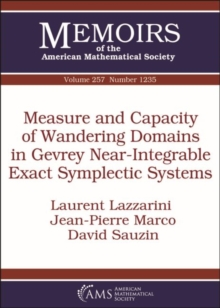Supporting your high street Find out how »
• My Account# Measure and Capacity of Wandering Domains in Gevrey Near-Integrable Exact Symplectic Systems Paperback / softback

## Part of the Memoirs of the American Mathematical Society series

#### Description

A wandering domain for a diffeomorphism $\Psi$ of $\mathbb A^n=T^*\mathbb T^n$ is an open connected set $W$ such that $\Psi ^k(W)\cap W=\emptyset$ for all $k\in \mathbb Z^*$.

The authors endow $\mathbb A^n$ with its usual exact symplectic structure.

An integrable diffeomorphism, i.e., the time-one map $\Phi ^h$ of a Hamiltonian $h: \mathbb A^n\to \mathbb R$ which depends only on the action variables, has no nonempty wandering domains. The aim of this paper is to estimate the size (measure and Gromov capacity) of wandering domains in the case of an exact symplectic perturbation of $\Phi ^h$, in the analytic or Gevrey category.

Upper estimates are related to Nekhoroshev theory; lower estimates are related to examples of Arnold diffusion.

This is a contribution to the quantitative Hamiltonian perturbation theory'' initiated in previous works on the optimality of long term stability estimates and diffusion times; the emphasis here is on discrete systems because this is the natural setting to study wandering domains.

#### Information

• Format: Paperback / softback
• Pages: 106 pages
• Publisher: American Mathematical Society
• Publication Date:
• Category: Calculus & mathematical analysis
• ISBN: 9781470434922

£84.50

£83.65£26.50

£26.15

£36.95

£16.35

£84.50

£82.85

£81.50

£79.85